# Difference between revisions of "Deadweight loss due to market power of sellers"

This page discusses a form of deadweight loss that occurs in the case that sellers have market power (i.e., they have some flexibility to set prices) but they are still restrained from price discrimination. The combination of market power and the inability to practice perfect price discrimination is crucial for this form of deadweight loss.

The core idea is that because of market power, sellers may price goods higher than they otherwise would, in order to reap greater profit margins per unit despite lower quantity of sales. As a result, some buyers are priced out. If the sellers had the flexibility to perfectly price discriminate, this situation would not occur: the seller would sell at the higher prices to those buyers who have higher reservation prices and at lower prices to those buyers who have lower reservation prices.

Situation Quantity traded Price Revenue Producer surplus = Profit (not accounting for fixed costs) Consumer surplus Total social surplus in diagram
Monopoly, with no ability to price discriminate Quantity point at which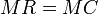$MR = MC$ and the$MC$ curve overtakes the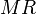$MR$ curve. Obtained by looking at the demand curve for the quantity.$PQ$, alternatively, equal to the area under the$MR$ curve up till the quantity choice.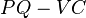$PQ - VC$, or equivalently, the signed area between the horizontal line for optimal price and the marginal cost curve.
In the picture above, this is the area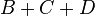$B + C + D$
The area between the market demand curve and the horizontal line for the price.
In the picture above, this is the area$A$.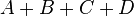$A + B + C + D$
Regulated monopoly, with externally enforced socially optimal price. Quantity point for intersection of market demand curve and marginal cost curve. Price point of intersection of demand curve and marginal cost curve.$PQ$, alternatively, equal to the area under the$MR$ curve up till the quantity choice.$PQ - VC$, or equivalently, the signed area between the horizontal line for socially optimal price and the marginal cost curve.
In the picture above, this is the area$C + D + F$
The area between the market demand curve and the horizontal line for the price.
In the picture above, this is the area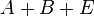$A + B + E$.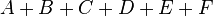$A + B + C + D + E + F$
Perfect price discriminating monopolistic firm Quantity point for intersection of market demand curve and marginal cost curve. Undefined, since the firm chooses different prices for different sales. The area under the market demand curve up till the chosen quantity of production.$PQ - VC$, or equivalently, the signed area between the horizontal line for socially optimal price and the marginal cost curve.
In the picture above, this could be as large as the area$A + B + C + D + E + F$, though it may not reach that extreme.$A + B + C + D + E + F$ minus the producer surplus. This could well be zero.$A + B + C + D + E + F$